I refer to the SAS documentation every day. Usually, I want information about SAS syntax and the statistical formulas and algorithms for various options and statements. Although I have bookmarked common documentation books and chapters, sometimes it is easier to perform an internet search to find information. I've discovered a combination of search terms that usually find the information I need with one search. The trick works well with the most popular search engines, Google and Bing, but I suspect it will work equally well with DuckDuckGo and other search engines.

The Rolling Stones famously sang, "You can't always get what you want. But if you try sometimes, well, you might find, you get what you need." With this internet search trick, I find that I get what I need most of the time.

To illustrate why these tips are useful, suppose you want to search for information about the CORRB option on the MODEL statement in PROC REG. You might go to your favorite search engine and type in "PROC REG" CORRB. The results include links to old SAS documentation (SAS 8.2 and 9.1), links to discussion forums (StackOverflow), and a regression tutorial from UCLA. If you want to bypass those other sources go directly to the most recent SAS documentation, read on.

### Two tips to improve your search for syntax and details

The key to searching the documentation is understanding how the SAS documentation is organized and how the documentation writers refer to syntax. Here are several tips for how to use an internet search engine to search the SAS documentation effectively:

• "SAS Help Center": Rather than search for a generic term like "SAS documentation," include the term "SAS Help Center," which is the name SAS gives to its online collection of documentation books. If you omit this term, you might find that your search engine returns results from older versions of SAS, such as SAS 9.2. To get the most current documentation, always start the search with "SAS Help Center".
• "XYZ Procedure": Although programmers colloquially refer to SAS procedures as "PROCs," the documentation system uses the term procedure. Thus, instead of searching for "PROC REG," search for "REG Procedure". Instead of searching for "PROC MEANS," search for "MEANS Procedure".

These two tips will go a long way to improving your ability to find the most recent documentation of SAS procedures. For example, try searching for the following terms:

• "SAS Help Center" "REG Procedure"
• "SAS Help Center" "MEANS Procedure"

Both searches result in links to the current documentation near the top of the search results. The links bring you to the syntax and details for the respective procedures. For me, the links typically resolve to the "SAS 9.4/Viya 3.5" version of the documentation, but you can easily change to more recent (or older!) versions of SAS by using the drop-down menu on the blue banner at the top of the web page.

You can, of course, restrict your search to the SAS documentation by using the site keyword, such as site:documentation.sas.com, but that technique does not ensure that you find the most recent documentation. I prefer using "SAS Help Center".

### Searching for statements or options

Two more tips:

• option: Include the word 'option' when searching for a procedure option. For example, if you are interested in the CORRB option on the MODEL statement in PROC REG, use the search terms option CORRB to the previous search. For the most general search criterion, do not use quotes around these terms.
• statement: Procedures typically support many statements. If you are an experienced SAS programmer, you probably know the names of the statements, such as the CLASS statement, MODEL statement, OUTPUT statement, and so forth. Add a search term such as "MODEL statement" to your search criterion.

### Searching for functions and subroutines

Sometimes I search for the documentation of functions or subroutines in the DATA step or in SAS/IML software. In those cases, append the word "function" or "call" to the end of the search string. For example, "SUM function" will find the documentation for the SUM function in both SAS/IML and the DATA step. "SVD call" will find the documentation for the SVD subroutine in SAS/IML.

### Summary

I often want to focus my search queries on the most recent SAS documentation. Adding "SAS Help Center" to my internet searches usually enables me to view the most recent documentation for SAS procedures and functions. I've included a few other tips that usually result in a direct link to procedures, statements, options, functions, and subroutines.

For more tips and suggestions about SAS documentation:

The post How to quickly find documentation for a SAS procedure appeared first on The DO Loop.When there are two equivalent ways to do something, I advocate choosing the one that is simpler and more efficient. Sometimes, I encounter a SAS program that simulates random numbers in a way that is neither simple nor efficient. This article demonstrates two improvements that you can make to your SAS code if you are simulating binary variables or categorical variables.

### Simulate a random binary variable

The following DATA step simulates N random binary (0 or 1) values for the X variable. The probability of generating a 1 is p=0.6 and the probability of generating a 0 is 0.4. Can you think of ways that this program can be simplified and improved?

```%let N = 8; %let seed = 54321;   data Binary1(drop=p); call streaminit(&seed); p = 0.6; do i = 1 to &N; u = rand("Uniform"); if (u < p) then x=1; else x=0; output; end; run;   proc print data=Binary1 noobs; run;```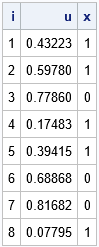The goal is to generate a 1 or a 0 for X. To accomplish this, the program generates a random uniform variate, u, which is in the interval (0, 1). If u < p, it assigns the value 1, otherwise it assigns the value 0.

Although the program is mathematically correct, the program can be simplified. It is not necessary for this program to generate and store the u variable. Yes, you can use the DROP statement to prevent the variable from appearing in the output data set, but a simpler way is to use the Bernoulli distribution to generate X directly. The Bernoulli(p) distribution generates a 1 with probability p and generates a 0 with probability 1-p. Thus, the following DATA step is equivalent to the first, but is both simpler and more efficient. Both programs generate the same random binary values.

```data Binary2(drop=p); call streaminit(&seed); p = 0.6; do i = 1 to &N; x = rand("Bernoulli", p); /* Bern(p) returns 1 with probability p */ output; end; run;   proc print data=Binary2 noobs; run;```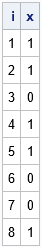### Simulate a random categorical variable

The following DATA step simulates N random categorical values for the X variable. If p = {0.1, 0.1, 0.2, 0.1, 0.3, 0.2} is a vector of probabilities, then the probability of generating the value i is p[i]. For example, the probability of generating a 3 is 0.2. Again, the program generates a random uniform variate and uses the cumulative probabilities ({0.1, 0.2, 0.4, 0.5, 0.8, 1}) as cutpoints to determine what value to assign to X, based on the value of u. (This is called the inverse CDF method.)

```/* p = {0.1, 0.1, 0.2, 0.1, 0.3, 0.2} */ /* Use the cumulative probability as cutpoints for assigning values to X */ %let c1 = 0.1; %let c2 = 0.2; %let c3 = 0.4; %let c4 = 0.5; %let c5 = 0.8; %let c6 = 1;   data Categorical1; call streaminit(&seed); do i = 1 to &N; u = rand("Uniform"); if (u <=&c1) then y=1; else if (u <=&c2) then y=2; else if (u <=&c3) then y=3; else if (u <=&c4) then y=4; else if (u <=&c5) then y=5; else y=6; output; end; run;   proc print data=Categorical1 noobs; run;```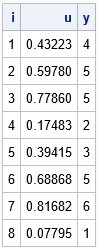If you want to generate more than six categories, this indirect method becomes untenable. Suppose you want to generate a categorical variable that has 100 categories. Do you really want to use a super-long IF-THEN/ELSE statement to assign the values of X based on some uniform variate, u? Of course not! Just as you can use the Bernoulli distribution to directly generate a random variable that has two levels, you can use the Table distribution to directly generate a random variable that has k levels, as follows:

```data Categorical2; call streaminit(&seed); array p _temporary_ (0.1, 0.1, 0.2, 0.1, 0.3, 0.2); do i = 1 to &N; x = rand("Table", of p[*]); /* Table(p) returns i with probability p[i] */ output; end;   proc print data=Categorical2 noobs; run;```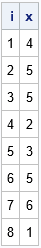### Summary

In summary, this article shows two tips for simulating discrete random variables:

1. Use the Bernoulli distribution to generate random binary variates.
2. Use the Table distribution to generate random categorical variates.

These distributions enable you to directly generate categorical values based on supplied probabilities. They are more efficient than the oft-used method of assigning values based on a uniform random variate.

The post Tips to simulate binary and categorical variables appeared first on The DO Loop.The Kronecker product (also called the direct product) is a binary operation that combines two matrices to form a new matrix. The Kronecker product appears in textbooks about the design of experiments and multivariate statistics. The Kronecker product seems intimidating at first, but often one of the matrices in the product has a special form, such as being a matrix of all 1s. In these special cases, the Kronecker product becomes much easier to understand. This article demonstrates some of the most common uses of the Kronecker product, including using it to concatenate matrices and to form block matrices.

### What is the Kronecker product?

The Kronecker product is best understood by thinking about block matrices. If A is a n x p matrix and B is a m x q matrix, then the Kronecker product A ⊗ B is a block matrix that is formed from blocks of B, where each block is multiplied by an element of A: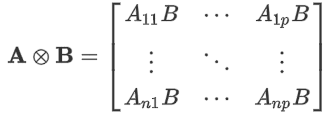The SAS/IML language uses the "at sign" (@) to represent the binary operator. Thus, the SAS/IML expression A @ B forms the Kronecker product A ⊗ B.

### The Kronecker product for vectors of 1s and special matrices

If A or B has a special form, the Kronecker product simplifies. First, consider only the case where A is a vector of all 1s or a special matrix. The following statements follow directly from the definition of the Kronecker product.

• If A is a row vector of all 1s, then A ⊗ B is the horizontal concatenation of p copies of B.
• If A is a column vector of all 1s, then A ⊗ B is the vertical concatenation of n copies of B.
• If A is a matrix of all 1s, then A ⊗ B is a block matrix that contains blocks of the matrix B.
• If A is the identity matrix, then A ⊗ B is a block diagonal matrix that contains copies of the matrix B along the diagonal.

Next, let A be an arbitrary matrix but consider only special forms for B:

• If B is a row vector of all 1s, then A ⊗ B contains q copies of the first column of A, followed by q copies of the second column of A, and so forth. I call this sequential replication of columns of A.
• If B is a column vector of all 1s, then A ⊗ B contains m copies of the first row of A, followed by m copies of the second row of A, and so forth. This is sequential replication of rows of A.
• If B is a matrix of all 1s, then A ⊗ B is a block matrix where the (i,j)th block is the constant matrix where all elements are A[i,j].
• If B is an identity matrix, then A ⊗ B is a block matrix where the (i,j)th block is the matrix A[i,j]*I.

The next sections show concrete examples of these eight ways to use the Kronecker product operator.

### Use the Kronecker product for horizonal or vertical concatenation

You can use the Kronecker product to perform horizontal or vertical concatenation. For example, the following SAS/IML program defines two vectors that contain only 1s. The vector w is a row vector and the vector h is a column vector. The program computes w ⊗ B and h ⊗ B for various choices of B. The ODS LAYOUT GRIDDED statement arranges the output in a grid.

```proc iml; /* specify RHS vectors */ w = {1 1}; /* w for 'wide' (row vector) */ h = {1, 1}; /* h for 'high' (col vector) */   /* specify data matrices */ r = {1 2 3}; /* row vector */ c = {1, 2, 3}; /* column vector */ x = {1 2 3, /* 2 x 3 matrix */ 4 5 6};   /******************************/ /* vector @ B : Concatenation */ /******************************/ wr = w @ r; /* horiz concat. Same as repeat(r, 1, ncol(w)) */ wx = w @ x; /* horiz concat. Same as repeat(x, 1, ncol(w)) */ hc = h @ c; /* vert concat. Same as repeat(c, nrow(h), 1) */ hx = h @ x; /* vert concat. Same as repeat(x, nrow(h), 1) */   ods layout gridded columns=2 advance=table; print wr[L='w@r'], wx[L='w@x'], hc[L='h@c'], hx[L='h@x']; ods layout end;```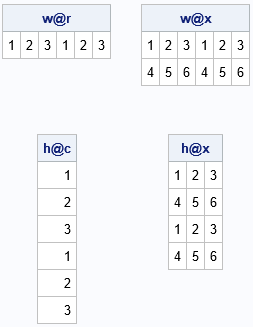The output indicates that the Kronecker product with w on the left results in horizontal concatenation. Using h on the left results in vertical concatenation.

### Use the Kronecker product for sequential replication

The previous section showed how to take a sequence of numbers such as {1,2,3} and repeat the entire sequence to form a new sequence such as {1,2,3,1,2,3}. But sometimes you want to repeat each element before repeating the next element. In other words, you might be interested in forming a sequence such as {1,1,2,2,3,3}. You can perform this kind of "sequential replication" by putting the data on the left and the vectors of 1s on the right, as follows:

```/***************************************/ /* A @ vector : Sequential replication */ /***************************************/ rw = r @ w; /* repeat cols sequentially */ xw = x @ w; ch = c @ h; /* repeat rows sequentially */ xh = x @ h;   ods layout gridded columns=2 advance=table; print rw[L='r@w'], xw[L='x@w'], ch[L='c@h'], xh[L='x@h']; ods layout end;```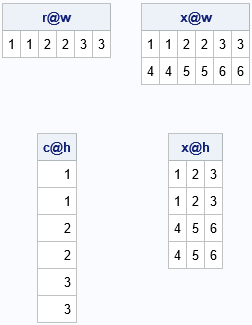It is worth mentioning that the second row of output is the transpose of the first row. That is because the transpose operation "distributes" over the Kronecker product operator. That is, for any matrices A and B, it is true that (A ⊗ B)` = A` ⊗ B`. Since r`=c and w`=h, then (r ⊗ w)` = (r` ⊗ w`) = c ⊗ h.

### Use the Kronecker product to construct block matrices

The Kronecker product is essentially an operation that forms block matrices. When one of the components is a vector of all 1s, then "forming a block matrix" is the same as concatenation. But if A is a binary matrix, then A ⊗ B is a block matrix that has the same structure as A, but each nonzero block is a copy of B.

Probably the most important subcase is that the matrix I(n) ⊗ B is a block diagonal matrix, where each diagonal block is a copy of B. The following list gives some other examples that use the identity or a constant matrix. Let I(n) be the n x k identity matrix and let J(n) be the n x n matrix of all 1s. Then

• I(n) ⊗ B is a block diagonal matrix with copies of B on the diagonal.
• J(n) ⊗ B is a block matrix with a copy of B in each block.
• A ⊗ I(n) is a block matrix where each block is a diagonal matrix.
• A ⊗ J(n) is a block matrix where each block is a constant matrix.
```Ix = I(2) @ x; /* diagonal block matrix */ Jx = J(2) @ x; /* 2 x 2 block matrix */ xI = x @ I(2); /* blocks of diagonal matrices */ xJ = x @ J(2); /* blocks of constant matrices */   ods layout gridded columns=2 advance=table; print Ix[L='I@x'], Jx[L='J@x'], xI[L='x@I'], xJ[L='x@J']; ods layout end;```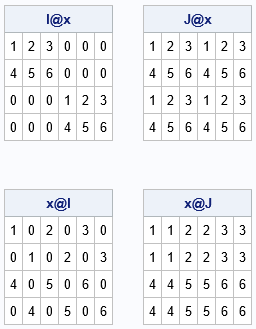### How is the Kronecker product used in statistics?

Recall that (mathematically) you can only add matrices that have the same dimensions. For example, you can't add a matrix and a vector. However, a common operation in multivariate statistics is to center a data matrix by subtracting the mean of each column from that column. If X is the data matrix, "mean(X)" is a row vector where the i_th element of the vector is the mean of the i_th column. The centering operation is sometimes informally written as "X – mean(X)," even though that expression does not make mathematical sense. Formally, you need to vertically concatenate the mean vector n times, where n is the number of rows in the data matrix. As we have seen, if h is a column vector of length n that contains all 1s, then mean(X) ⊗ h is a matrix and the expression X – mean(X) ⊗ h is a proper mathematical expression that indicates the centering operation.

As described in a previous article, in the early days of SAS, statistical programmers had to use Kronecker product operator to ensure that all matrix operations were mathematically correct. But that changed in SAS version 8 (circa 1999) when the IML language introduced a shorthand notation that makes it easier to perform row and column operations. Back in the "old days," you saw lots of programs that used the Kronecker operator like this:

```/* read data matrix into X */ use Sashelp.Class; read all var _num_ into X; close; mean = x[:,]; /* row vector of means for each column */ h = j(nrow(X), 1, 1); /* column vector */ CenterX = X - mean @ h; /* subtract matrices of same dimension */```

The modern SAS/IML programmer will typically use the shorthand notation to avoid the Kronecker product:

`CenterX = X - mean; /* modern code: Subtract the row vector from each row of X */`

Although you no longer need to use the Kronecker product for basic centering operations, the Kronecker operator is still useful for other tasks, such as building block matrices and concatenation. Block diagonal matrices are used in mixed models of longitudinal data (Chapter 12 of Wicklin, 2013).

### Summary

This article shows eight ways that you can use the Kronecker product. The Kronecker operator enables you to perform horizontal and vertical concatenation, perform sequential repetition of rows and columns, and build block matrices with special structure. You can use this article as a "cheat sheet" to remind you about some common uses of the Kronecker operator.

The post 8 ways to use the Kronecker product appeared first on The DO Loop.I previously wrote about the advantages of adding horizontal and vertical reference lines to a graph. You can also add a diagonal reference line to a graph. The SGPLOT procedure in SAS supports two primary ways to add a diagonal reference line:

• The LINEPARM statement enables you to specify a point on the line and the slope of the line. The line extends the whole length of the graph.
• The VECTOR statement enables you to add a line segment or an arrow. You specify the initial and final points for the line segment.

This article shows how to use the LINEPARM and VECTOR statements in PROC SGPLOT. I'll call these lines "diagonal lines," but the LINEPARM and VECTOR statement can also create horizontal lines (slope=0) or vertical lines (a missing value for the slope).

### A basic reference line

The LINEPARM statement in PROC SGPLOT is easy to use. For a single line, you use the X= and Y= keywords to specify a point that the line passes through. You use the SLOPE= option to specify the slope of the line.

The most common diagonal reference line is the identity line y = x. This is useful for comparing data before and after an intervention. For example, the following SAS DATA step specifies the weights for 15 women in an eight-week weight loss program. You can plot each woman's weight before and after the program. It is useful to add the identity line so that you can see at a glance whether each woman lost weight or not. The weights of women who lost weight appear below the identity line. Women who did not lose weight appear on or above the line, as shown in the following:

```data Weight; input Before After @@; label Before="Baseline Weight (kg)" After="Final Weight (kg)"; datalines; 68 63 82 77 100 98 71 72 79 75 109 100 93 89 88 84 78 74 69 69 96 98 91 89 86 81 80 75 68 65 run;   title "lineparm x=0 y=0 slope=1"; proc sgplot data=Weight noautolegend; scatter x=Before y=After; lineparm x=0 y=0 slope=1; /* identity line passes through (0, 0) and has slope=1 */ xaxis grid; yaxis grid; run;```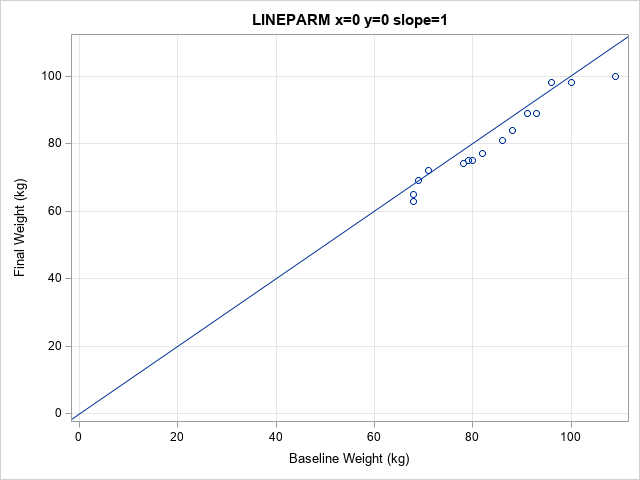In this graph, most markers are below the line, which indicates that most women lost weight. Notice, however, that most of the markers are squashed into the upper right portion of the graph. The graph shows origin (0, 0) because I used X=0 Y=0 to specify a point on the line. You can eliminate the wasted space by specifying the CLIP option on the LINEPARM statement. The CLIP option tells PROC SGPLOT to ignore the LINEPARM statement when setting the range for the axes. This is shown in the following call:

```title "The CLIP Option for the LINEPARM Statement"; proc sgplot data=Weight noautolegend; scatter x=Before y=After; lineparm x=0 y=0 slope=1 / CLIP; /* identity line passes through (0, 0) and has slope=1 */ xaxis grid; yaxis grid; /* use MAX=110 if you want to see the line when x=110 */ run;```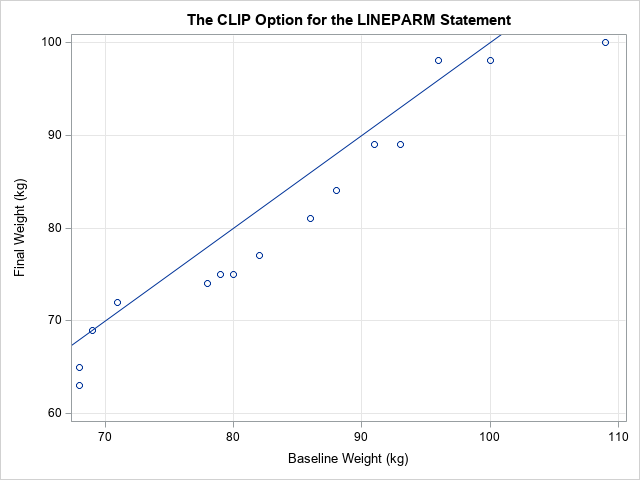This graph is preferable in most situations. The range of the axes are based only on the data (not the line), so you can see the data more clearly.

### Multiple diagonal lines

If you want to display multiple diagonal lines, you can use multiple LINEPARM statements, or you can use one LINEPARM statement and specify a variable in the data set that contains values for the points and slopes of each line.

For example, suppose you want to display tangent lines to the curve y=f(x) at several locations along the curve. As you learned in calculus, the equation of the tangent line at (x0, y0) is y = y0 + m(x-x0), where m = f`(x0) is the slope of the curve at x0. the following program generate points on the graph of y = exp(x/2) and computes the slope of the curve for x0 = 0, 1, 2, and 3.

```/* First data set: points along a curve */ data Func; do x = 0 to 4 by 0.01; y = exp(x/2); output; end; run;   /* Second data set: points and slopes for the tangent lines at four locations */ data Lines; do x0 = 0 to 3; y0 = exp(x0/2); /* y0 = f(x0) */ Slope = (1/2)*y0; /* m - f`(x0) */ ID + 1; output; end; run;   data F1; set Func Lines; /* concatenate the data sets */ run;   title "Exponential Function and Tangent Lines"; proc sgplot data=F1 noautolegend; series x=x y=y / lineattrs=(thickness=2) curvelabel="y = exp(x/2)"; /* curve */ lineparm x=x0 y=y0 slope=Slope / clip group=ID; /* tangent lines */ scatter x=x0 y=y0 / group=ID markerattrs=(symbol=CircleFilled size=8); /* points of tangency */ run;```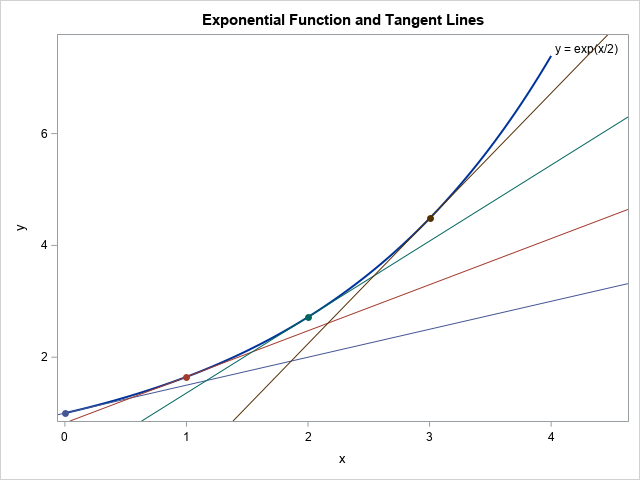The graph shows four tangent lines. The points and slopes that determine the tangent lines are in the Lines data set. Those parameters are concatenated with the data for the curve so that the curve and the tangent lines can be drawn on a single graph. The LINEPARM statement reads the X0, Y0, and Slope variables and uses the values to draw the lines.

### Vectors and line segments

As you can see from the graphs in the previous section, the LINEPARM statement draws a line that spans the full length of the graph. Sometimes you might want to display a line segment instead. The VECTOR statement in PROC SGPLOT enables you to draw a line segment that starts at an initial point, (x0, y0), and ends at a final point, (xf, yf). By default, the VECTOR statement draws an arrow at the end point.

You can use the VECTOR statement to draw unit tangent vectors to the curve. If you take a unit step in the horizontal direction, then the tangent vector is (1, f`(x0)), so you can divide by the vector norm (which is sqrt(1 + (f`(x0))2)) to obtain a unit tangent vector.

```data Vectors; do x0 = 0 to 3; y0 = exp(x0/2); slope = (1/2)*y0; dx = 1 / sqrt(1 + slope**2); /* choose dx to create a unit tangent vector */ xf = x0 + dx; yf = y0 + slope*dx; ID + 1; output; end; run;   data F2; set Func Vectors; run;   title "Exponential Function and Tangent Vectors"; proc sgplot data=F2 noautolegend; series x=x y=y / curvelabel="y = exp(x/2)"; vector x=xf y=yf / xorigin=x0 yorigin=y0 group=ID; scatter x=x0 y=y0 / group=ID markerattrs=(symbol=CircleFilled size=8); xaxis grid; run;```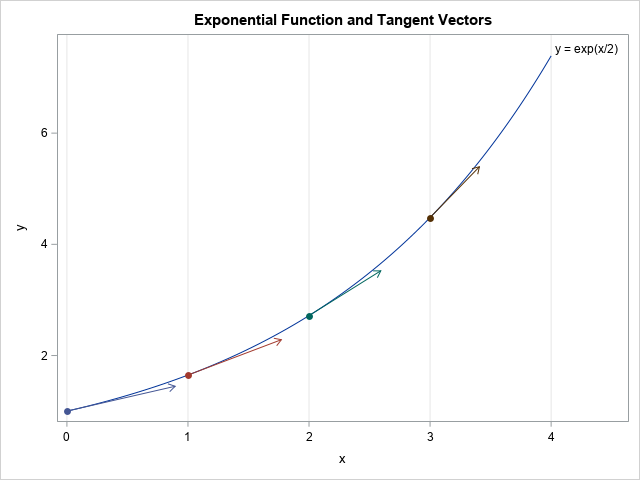### Summary

In summary, you can use the LINEPARM statement in PROC SGPLOT to add diagonal lines to a graph. The lines span the graph from border to border. If you want to plot a line segment or an arrow, you can use the VECTOR statement.

The post Add diagonal reference lines to SAS graphs: The LINEPARM and VECTOR statements appeared first on The DO Loop.Data tell a story. A purpose of data visualization is to convey that story to the reader in a clear and impactful way. Sometimes you can let the data "speak for themselves" in an unadorned graphic, but sometimes it is helpful to add reference lines to a graph to emphasize key features of the data.

This article discusses the REFLINE statement in PROC SGPLOT in SAS. This is a statement that I use daily. This article provides multiple "Getting Started" examples that show how to use the REFLINE statement to improve your graphs. Examples include:

• Display a reference line at a value such as a mean or median
• Add labels to a reference line
• Display normal ranges for measurements
• Use reference lines for a categorical variable on a discrete axis

### Basic reference lines

The REFLINE statement in PROC SGPLOT is easy to use. You can specify one or more values (separated by spaces) or you can specify a variable in the data set that contains the values at which to display the reference lines. You then use the AXIS=X or AXIS=Y option to specify which axis the reference lines are for. The reference lines are perpendicular to the axis.

A simple use of a reference line is to indicate a reference value on a histogram. For example, a healthy total cholesterol level is less than 200 mg/dL. A "borderline" (or moderately elevated) cholesterol level is between 200 and 240 mg/dL. A cholesterol level that is 240 or more is considered high. The Sashelp.Heart data set contains cholesterol and blood pressure information for patients in a heart study. The following histogram shows the distribution of cholesterol values for 5,195 subjects. You can use reference lines to indicate good, borderline, and high cholesterol.

```data Heart; set sashelp.Heart(where=(Cholesterol<400)); keep Cholesterol Systolic; run;   proc sgplot data=Heart; histogram Cholesterol; refline 200 240 / axis=x lineattrs=(thickness=3 color=darkred pattern=dash); /* Note: Order matters. Put REFLINE stmt first if you want it behind the bars */ run;```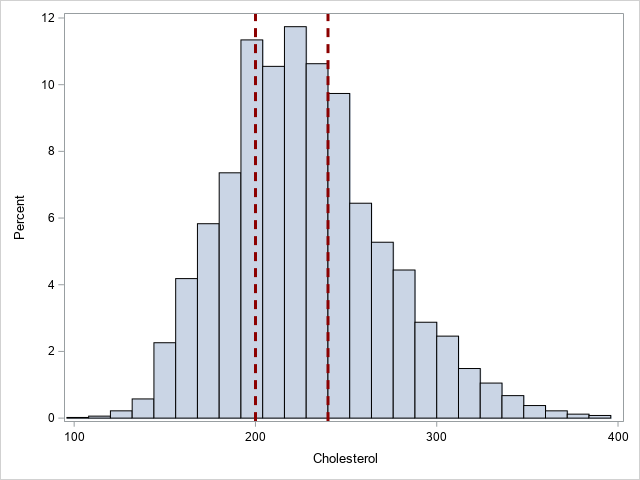In this example, I used the optional LINEATTRS= option to show how to change the color, line pattern, and thickness of the reference lines.

### Reference lines with labels

If you might want to add a label to the reference lines, you can use the LABEL= option to specify one or more labels. You can use the LABELLOC= option to put the label inside or outside the data area of the graph. I like "outside" (the default) because then the line does not interfere with the label. You can use the LABELPOS= option to specify whether the label is displayed at the top or bottom (for a vertical reference line) or at the left or right (for a horizontal reference line). The following example adds labels to the previous example.

```proc sgplot data=Heart; histogram Cholesterol; refline 200 240 / axis=x lineattrs=(thickness=3 color=darkred pattern=dash) label=("Borderline" "High"); /* OPT: labelloc=inside labelpos=max; */ run;```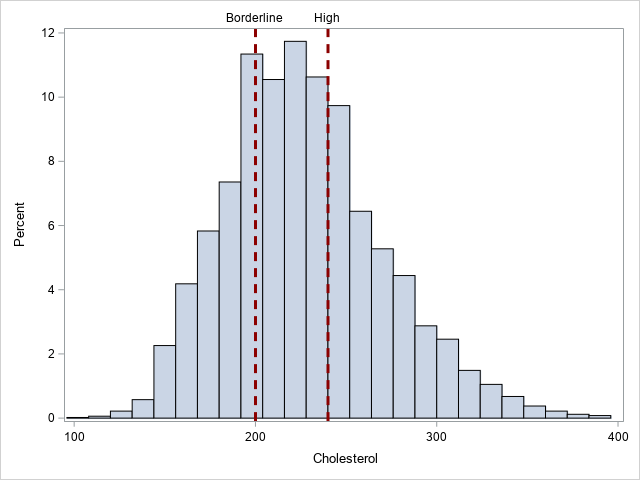### Reference lines at computed locations

Sometimes the reference values are the result of a computation. The REFLINE values and the LABEL= option can come from variables in a SAS data set. For multiple values, you probably want to arrange the values in "long form."

A good example is displaying descriptive statistics such as a mean, median, and percentiles. The following call to PROC MEANS computes three statistics for the Cholesterol variable: the median, the 25th percentile, and the 75th percentile. The output from PROC MEANS is one row and three columns, so I use PROC TRANSPOSE to convert the data set into long form, as follows:

```/* create a data set of statistics */ proc means data=Heart Median P25 P75; var Cholesterol; output out=MeansOut(drop=_TYPE_ _FREQ_) median=Median P25=P25 P75=P75; run;   proc transpose data=MeansOut Name=Stat out=Stats(rename=(Col1=CholValue)); run;   proc print data=Stats noobs; run;```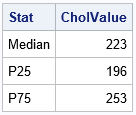You can append the statistics to the original data set and use PROC SGPLOT to create a histogram with reference lines that display the computed percentiles.

```data HeartChol; set Heart Stats; run;   proc sgplot data=HeartChol; histogram Cholesterol; refline CholValue / axis=x label=Stat lineattrs=GraphData2(thickness=3); run;```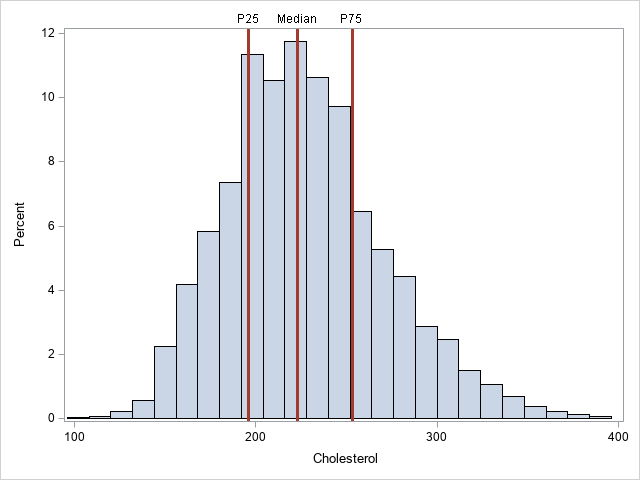In this example, I used the LINEATTRS=GRAPHDATA2 option to assign the style attributes of the lines. I used the THICKNESS= suboption to override the default thickness.

### Reference lines for 2-D plots

You can also add reference lines to one or both axes of a two-dimensional plot such as a scatter plot, heat map, or contour plot. The following graph shows a heat map of the cholesterol and systolic blood pressure values for more than 5,000 patients. The reference lines show clinical values for normal, slightly high, and high levels of both variables:

```title "Clinical Ranges of Systolic Blood Pressure and Cholesterol"; proc sgplot data=HeartStats; heatmap x=Cholesterol y=Systolic / colormodel=(CXDEEBF7 CX9ECAE1 CX3182BD ); refline 200 240 / axis=x label=('Borderline' 'High') lineattrs=GraphData2; refline 120 130 / axis=y label=('Elevated' 'Hypertensive') lineattrs=GraphData2; gradlegend / position=bottom; run;```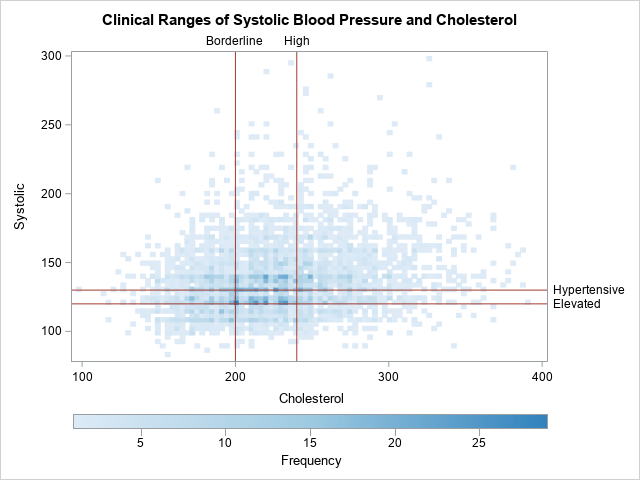### Reference lines for a discrete axis

You can also display reference lines on a discrete axis, although it is not common. One application that I can think of is displaying an expected value for a discrete probability distribution. Another application is simply drawing a line that separates one set of categories from another. In the following example, I use a reference line to indicate a fiscal year. Notice the following:

• If the categorical variable has a format, you need to specify the formatted value.
• By default, the reference line will be in the middle of the category. You can use the DISCRETEOFFSET= option and a value in the interval [-0.5, 0.5] to move the line left or right of center. Positive values move the line to the right; negative values move the line to the left. In the example, DISCRETEOFFSET=0.5 moves the line between the reference category and its neighbor to the right.
• The REFLINE statement supports a SPLITCHAR= option that you can use to split a long label across multiple lines.
```data Revenue; input Quarter Date9. Revenue; label Revenue = "Revenue (millions)"; format Quarter YYQ4.; datalines; 01Sep2018 1.5 01Dec2018 2.7 01Mar2019 1.2 01Jun2019 1.6 01Sep2019 1.4 01Dec2019 2.8 01Mar2020 0.8 ;   title "Quarterly Revenue for ABC Corp"; proc sgplot data=Revenue; vbar Quarter/ response=Revenue; /* for a discrete variable, specified the formatted value */ refline "19Q2" / axis=x discreteoffset=0.5 /* move ref line to right */ labelloc=inside label="Fiscal /Year " splitchar="/"; /* split label */ xaxis discreteorder=data; yaxis grid offsetmax=0.1; run;```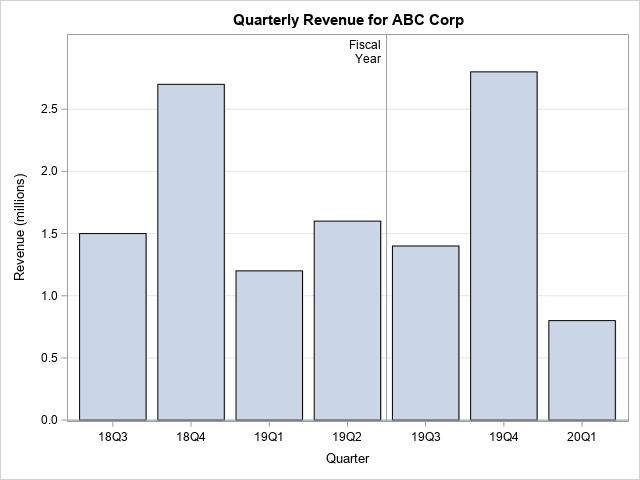### Getting fancy with reference lines

Because you can control the thickness of the reference lines, you can use them for many purposes. Sanjay Matange shows two creative uses for reference lines for a discrete axis:

### Summary

This article shows several ways to use the REFLINE statement in PROC SGPLOT to add information to your graphs. You can display a line to indicate a reference value or a sample statistic. You can display labels for reference lines. You can even use reference lines for a categorical variable on a discrete axis. Reference lines are a powerful way to enhance your graphs.

The post Add horizontal and vertical reference lines to SAS graphs: The REFLINE statement appeared first on The DO Loop.Recoding variables can be tedious, but it is often a necessary part of data analysis. Almost every SAS programmer has written a DATA step that uses IF-THEN/ELSE logic or the SELECT-WHEN statements to recode variables. Although creating a new variable is effective, it is also inefficient because you have to create a new data set that contains the new variable. For large data sets, this is wasteful: most of the data remain the same; only the recoded variables are different.

There is an alternative approach: You can use PROC FORMAT in Base SAS to define a custom SAS format. When you use PROC FORMAT, the data are never changed, but all the SAS reports and analyses can display the formatted values instead of the raw data values. You can use the same format for multiple data sets. You can even define multiple formats to analyze the same variable in multiple ways.

### An example of using a format to recode a variable

In the simplest situation, a recoding of a variable converts each raw value to an easier-to-interpret value. For example, suppose that the gender variable for patients is recorded as a binary 0/1 variable. This is a terrible choice because it is not clear whether 0 represents males or females. The following example shows the typical IF-THEN logic for recoding 0 as "Female" and 1 as "Male" by creating a new data set and a new variable:

```/* original data: Gender is binary variable, which is hard to understand! */ data Have; input Gender @@; datalines; 1 0 0 0 1 1 0 0 . 1 1 0 0 0 0 1 1 1 . 1 1 ;   /* Recode by using IF-THEN or SELECT-WHEN. This can be inefficient. */ data HaveRecode; set Have; /* use IF-THEN logic to recode gender */ length Gender_Recode \$6; if Gender=0 then Gender_Recode = "Female"; else if Gender=1 then Gender_Recode = "Male"; else Gender_Recode = " "; run;   proc freq data=HaveRecode; tables Gender_Recode Gender; run;```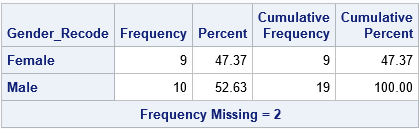The table for the Gender_Recode variable is shown. The data, which was originally coded as a binary indicator variable, has been duplicated by creating a character variable that contains the same information but is more understandable. Of course, now you have to use the new variable name to analyze the recoded data. If you have already written programs that refer to the Gender variable, you have to update the programs to use the new variable name. Yuck!

A more efficient choice is to use a custom-defined format. The beauty of using a format is that you do not have to change the data. Instead, you simply define a format that changes the way that the data are used and displayed in SAS procedures. (A data view is a third alternative, but formats have additional advantages.)

You can define the following format (called GenderFmt.), which displays the gender data as "Female" and "Male" without modifying the data set:

```/* use a format to recode gender */ proc format; value GenderFmt 0 = "Female" 1 = "Male" other = " "; run;   /* apply the format to original data; no need to create new data set */ proc freq data=Have; format Gender GenderFmt.; /* the name of the format includes a period */ tables Gender; run;```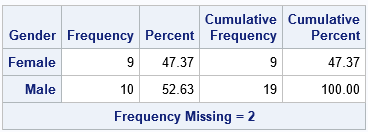Notice that the analysis is run on the original data and use the original variable name. No additional data sets, views, or variables are created.

### Use a format to recode a character variable

Did you know that you can use PROC FORMAT to define formats for character variables? Formats for character variables are used less often than formats for numeric variables, but the syntax is similar. The main difference is that the name of a character format starts with the '\$' symbol.

In addition to recoding the values of a categorical variable, formats are useful because they enable you to merge or combine categories by defining a many-to-one mapping. For example, the following character format recodes values of the TYPE variable and also combines the 'SUV' and 'Wagon' categories into a single category. Although it is not needed for this example, notice that the format also includes an 'Other' category, which can be used to combine small groups. The 'Other' category will also handle invalid data.

```/* Create sample data from Sashelp.Cars. Exclude hybrids. Optionally sort the data */ proc sort data=sashelp.cars(where=(Type^='Hybrid')) out=Cars; by MPG_City; run;   proc format; value \$CarTypeFmt 'Sedan' = 'Family Car' 'Sports' = 'Sports Car' 'SUV','Wagon' = 'Big Car' 'Truck' = 'Truck' Other = 'Other'; run;   proc freq data=Cars; format Type \$CarTypeFmt.; /* the name the format includes a period at the end */ tables Type; run;```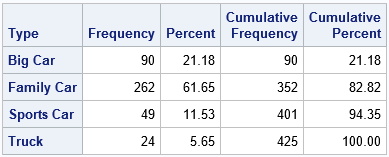Using a format enables you to analyze the original data (omit the FORMAT statement) or apply the format (include the FORMAT statement). You can even define multiple formats if you want to slice and dice the data in various ways.

### Use a format to bin numeric variables

One of my favorite SAS tricks is to use a format to bin numeric variables into categories. In the following example, the MPG_City variable is used to group vehicles into four categories based on how fuel-efficient the vehicles are. You can use this format to perform any computation that requires a classification variable. The example shows a two-way frequency analysis of the two variables for which we have defined custom formats:

```proc format; value MPGFmt low -< 15 = "Gas Guzzler" /* < 15 */ 15 -< 20 = "Not Good" /* [ 15, 20) */ 20 -< 25 = "Good" /* [ 20, 25) */ 25 - high = "Great"; /* > 25 */ run;   proc freq data=Cars order=data; format MPG_City MPGFmt. Type \$CarTypeFmt.; tables MPG_City * Type / nocol norow nopercent; run;```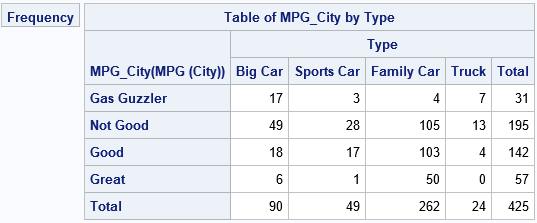### Store and retrieve formats

Formats are stored in a catalog, which is stored separately from the data. By default, SAS stores the formats in a catalog named WORK.FORMATS. Like everything else stored in WORK, that catalog will vanish when you end the SAS session. Therefore, you need to store the formats to a permanent libref if you want to reuse the formats across SAS session.

SAS supports several features that help you to maintain a permanent library of formats. Here are two facts about format catalogs:

• You can use the LIBRARY= option on the PROC FORMAT statement to specify a libref in which to store the format catalog. By default, the catalog will be named FORMATS.
• SAS maintains a list of librefs to search through to find formats. By default, it looks in WORK and a special libref named LIBRARY.

These facts imply that you can do two simple things to create a permanent library of formats. First, define a permanent libref named LIBRARY (the name is important!) that will contain your catalog of formats. Second, specify the LIBRARY=LIBRARY option when you define the format, as follows:

```libname library "C:/MyFormats"; /* the libref 'LIBRARY' has special significance! */ proc format library=library; /* adds format to the permanent catalog LIBRARY.FORMATS */ value \$CarTypeFmt 'Sedan' = 'Family Car' 'Sports' = 'Sports Car' 'SUV','Wagon' = 'Big Car' 'Truck' = 'Truck' Other = 'Other'; run;```

When you start a new SAS session, you will need to define the LIBRARY libref again if you want to access the formats. For convenience, many people put the LIBNAME statement in their AutoExec.sas file. Because SAS searches for formats in the LIBRARY.FORMATS catalog, SAS will automatically find the \$CarTypeFmt. format.

SAS provides many other options for storing formats and for specifying the search locations for formats. For details, see the SAS usage note "How can I permanently store and use formats that I have created?" or John Ladd's 2012 paper, "Yes, We Can... Save SAS Formats."

### Summary

In summary, if you need to recode data, custom-defined formats provide an easy alternative to physically changing the data. This article discusses five advantages to using formats to recode data:

• The data do not change. You can use the original variable names in the analyses.
• You can apply formats to both character and numerical variables.
• You can use formats to merge categories and to bin numeric variables.
• You apply a format to multiple variables in multiple data sets.
• You can save formats in a permanent libref and use them across SAS sessions.

The post 5 reasons to use PROC FORMAT to recode variables in SAS appeared first on The DO Loop.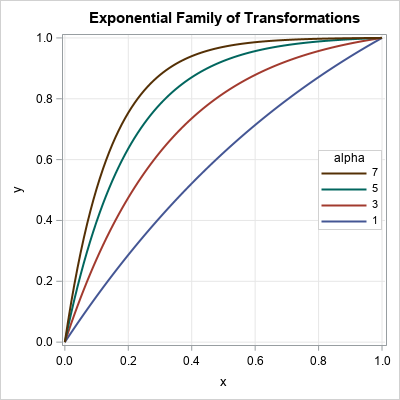A family of curves is generated by an equation that has one or more parameters. To visualize the family, you might want to display a graph that overlays four of five curves that have different parameter values, as shown to the right. The graph shows members of a family of exponential transformations of the form
f(x; α) = (1 – exp(-α x)) / (1 – exp(-α))
for α > 0 and x ∈ [0, 1]. This graph enables you to see how the parameter affects the shape of the curve. For example, for small values of the parameter, α, the transformation is close to the identity transformation. For larger values of α, the nonlinear transformation stretches intervals near x=0 and compresses intervals near x=1.

Here's a tip for creating a graph like this in SAS. Generate the data in "long format" and use the GROUP= option on the SERIES statement in PROC SGPLOT to plot the curves and control their attributes. The long format and the GROUP= option make it easy to visualize the family of curves.

### A family of exponential transformations

I recently read a technical article that used the exponential family given above. The authors introduced the family and stated that they would use α = 8 in their paper. Although I could determine in my head that the function is monotonically increasing on [0, 1] and f(0)=0 and f(1)=1, I had no idea what the transformation looked like for α = 8. However, it is easy to use SAS to generate members of the family for different values of α and overlay the curves:

```data ExpTransform; do alpha = 1 to 7 by 2; /* parameters in the outer loop */ do x = 0 to 1 by 0.01; /* domain of function */ y = (1-exp(-alpha*x)) / (1 - exp(-alpha)); /* f(x; alpha) on domain */ output; end; end; run;   /* Use ODS GRAPHICS / ATTRPRIORITY=NONE if you want to force the line attributes to vary in the HTML destination. */ ods graphics / width=400px height=400px; title "Exponential Family of Transformations"; proc sgplot data=ExpTransform; series x=x y=y / group=alpha lineattrs=(thickness=2); keylegend / location=inside position=E across=1 opaque sortorder=reverseauto; xaxis grid; yaxis grid; run;```

The graph is shown at the top of this article. The best way to create this graph is to generate the points in the long-data format because:

• The outer loop controls the values of the parameters and how many curves are drawn. You can use a DO loop to generate evenly spaced parameters or specify an arbitrary sequence of parameters by using the syntax
DO alpha = 1, 3, 6, 10;
• The domain of the curve might depend on the parameter value. As shown in the next section, you might want to use a different set of points for each curve.
• You can use the GROUP= option and the KEYLEGEND statement in PROC SGPLOT to visualize the family of curves.

### Visualize a two-parameter family of curves

You can use the same ideas and syntax to plot a two-parameter family of curves. For example, you might want to visualize the density of the Beta distribution for representative values of the shape parameters, a and b. The Wikipedia article about the Beta distribution uses five pairs of (a, b) values; I've used the same values in the following SAS program:

```data BetaDist; array alpha _temporary_ (0.5 5 1 2 2); array beta  _temporary_ (0.5 1 3 2 5); do i = 1 to dim(alpha); /* parameters in the outer loop */ a = alpha[i]; b = beta[i]; Params = catt("a=", a, "; b=", b); /* concatenate parameters */ do x = 0 to 0.99 by 0.01; pdf = pdf("Beta", x, a, b); /* evaluate the Beta(x; a, b) density */ if pdf < 2.5 then output; /* exclude large values */ end; end; run;   ods graphics / reset; title "Probability Density of the Beta(a, b) Distribution"; proc sgplot data=BetaDist; label pdf="Density"; series x=x y=pdf / group=Params lineattrs=(thickness=2); keylegend / position=right; xaxis grid; yaxis grid; run;```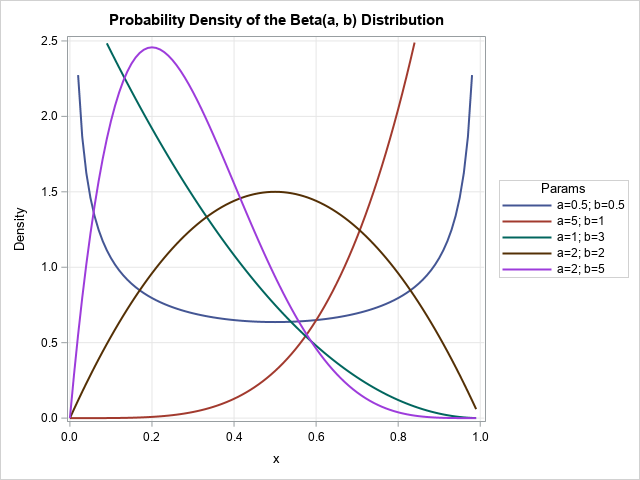The resulting graph gives a good overview of how the parameters in the Beta distribution affect the shape of the probability density function. The program uses a few tricks:

• The parameters are stored in arrays. The program loops over the number of parameters.
• A SAS concatenation functions concatenate the parameters into a string that identifies each curve. The CAT, CATS, CATT, and CATX functions are powerful and useful!
• For this family, several curves are unbounded. The program caps the maximum vertical value of the graph at 2.5.
• Although it is not obvious, some of the curves are drawn by using 100 points whereas others use fewer points. This is an advantage of using the long format.

In summary, you can use PROC SGPLOT to visualize a family of curves. The task is easiest when you generate the points along each curve in the "long format." The long format is easier to work with than the "wide format" in which each curve is stored in a separate Y variable. When the curve values are in long form, you can use the GROUP= option on the SERIES statement to create an effective visualization by using a small number of statements.

The post Plot a family of curves in SAS appeared first on The DO Loop.A SAS programmer posted an interesting question on a SAS discussion forum. The programmer wanted to iterate over hundreds of SAS data sets, read in all the character variables, and then do some analysis. However, not every data set contains character variables, and SAS complains when you ask it to read the character variables in a data set that contains only numeric variables.

The programmer wanted to use PROC IML to solve the problem, but the issue also occurs in the SAS DATA step. The following program creates three data sets. Two of them (AllChar and Mixed) contain at least one character variable. The third data set (AllNum) does not contain any character variables. For the third data set, an error occurs if you try to use the KEEP=_CHARACTER_ data set option, as shown in the following example:

```data AllNum; x=1; y=2; z=3; run; data AllChar; A='ABC'; B='XYZW'; run; data Mixed; name='Joe'; sex='M'; Height=1.8; Weight=81; treatment='Placebo'; run;   /* try to use DROP=_CHARACTER_ to exclude numeric variables */ data KeepTheChar; set AllNum(keep=_CHARACTER_); /* ERROR when no character variables in the data set */ run;```
` ERROR: The variable _CHARACTER_ in the DROP, KEEP, or RENAME list has never been referenced.`

The same problem occurs in PROC IML if you try to read character variables when none exist:

```proc iml; use AllNum; read all var _CHAR_ into X; /* ERROR when no character variables in the data set */ close;```
` ERROR: No character variables in the data set.`

There are at least two ways to handle this situation:

1. In both Base SAS and SAS/IML, you can use dictionary tables to determine in advance which data sets contain at least one character variable. You can then read only those data set.
2. In SAS/IML, you can read all variables into a table, then extract the character variables into a matrix for further processing.

Of course, the same ideas apply if you want to read only numeric variables and you encounter a data set that does not contain any numeric variables.

If you have ever been to a SAS conference, you know that DICTIONARY tables are a favorite topic for SAS programmers. DICTIONARY tables are read-only tables that provide information about the state of the SAS session, including libraries, data sets, variables, and system options. You can access them directly by using PROC SQL. If you want to access the information in the DATA steps or other procedures (like PROC IML), you can use special data views in SASHELP. In particular, the Sashelp.VColumn view provides information about variables in SAS data set and is often used to find data sets that contain certain variable names. (See the references at the end of this article for more information about DICTIONARY tables.)

The following SAS/IML program uses the Sashelp.VColumn to find out which data sets contain at least one character variable:

```proc iml; /* Solution 1: Use dictionary table sashelp.vcolumn */ /* Find data sets in WORK that have AT LEAST ONE character variable */ use sashelp.vcolumn(where=(libname="WORK" & memtype='DATA' & type='char'); /* read only CHAR variables */ read all var {memname name}; /* memname=data set name; name=name of character variable */ close;   /* loop over data sets. If a set contains at least one character variable, process it */ dsName = {'AllNum' 'AllChar' 'Mixed'}; /* names of potential data sets */ do i = 1 to ncol(dsName); idx = loc(memname = upcase(dsName[i])); /* is data set on the has-character-variable list? */ /* for demo, print whether data set has character variables */ msg = "The data set " + (dsName[i]) + " contains " + char(ncol(idx)) + " character variables."; print msg;   if ncol(idx)>0 then do; /* the data set exists and has character vars */ charVars = name[idx]; /* get the names of the character vars */ use (dsName[i]); /* open the data set for reading */ read all var charVars into X; /* read character variables (always succeeds) */ close; /* process the data */ end; end;```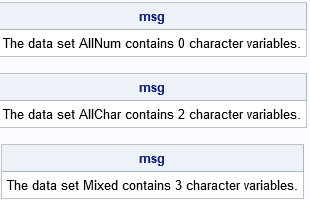The output shows that you can use the DICTIONARY tables to determine which data sets have at least one character variable. You can then use the USE/READ statements in PROC IML to read the character variables and process the data however you wish. As mentioned previously, this technique can also be used in PROC SQL and the DATA step.

### Use SAS/IML tables to find character variables

The previous section is very efficient because only character variables are ever read into SAS/IML matrices. However, there might be situations when you want to process character variables (if they exist) and then later process numerical variables (if they exist). Although a SAS/IML matrix contains only one data type (either all numeric or all character), you can read mixed-type data into a SAS/IML table, which supports both numeric and character variables. You can then use the TableIsVarNumeric function to generate a binary indicator variable that tells you which variables in the data are numeric and which are character, as follows:

```/* Solution 2: Read all data into a table. Use the TableIsVarNumeric function to determine which variables are numeric and which are character. */ dsName = {'AllNum' 'AllChar' 'Mixed'}; /* names of potential data sets */ do i = 1 to ncol(dsName); /* for each data set... */ T = TableCreateFromDataset("WORK", dsName[i]); /* read all variables into a table */ numerInd = TableIsVarNumeric(T); /* binary indicator vector for numeric vars */ charInd = ^numerInd; /* binary indicator vector for character vars */ numCharVars = sum(charInd); /* count of character variables in this data set */ msg = "The data set " + (dsName[i]) + " contains " + char(numCharVars) + " character variables."; print msg; if numCharVars > 0 then do; X = TableGetVarData(T, loc(charInd)); /* extract the character variables into X */ /* process the data */ end; /* optionally process the numeric data */ numNumerVars = sum(numerInd); /* count of numeric variables in this data set */ /* etc */ end;```

The output is identical to the output in the previous section.

### Summary

In summary, this article discusses a programmer who wants to iterate over many SAS data sets and process only character variables. However, some of the data sets do not have any character variables! This article shows two methods for dealing with this situation: DICTIONARY tables (available through Sashelp views) or SAS/IML tables. The first method is also available in Base SAS.

Of course, you can also use this trick to read all numeric variables when some of the data sets might not have any numeric variable. I've previously written about how to read all numeric variables into a SAS/IML matrix by using the _ALL_ keyword. If the data set contains both numeric and character variables, then only the numeric variables are read.

### References

The post How to detect SAS data sets that contain (or do not contain) character variables appeared first on The DO Loop.An ROC curve graphically summarizes the tradeoff between true positives and true negatives for a rule or model that predicts a binary response variable. An ROC curve is a parametric curve that is constructed by varying the cutpoint value at which estimated probabilities are considered to predict the binary event. Most SAS data analysts know that you can fit a logistic model in PROC LOGISTIC and create an ROC curve for that model, but did you know that PROC LOGISTIC enables you to create and compare ROC curves for ANY vector of predicted probabilities regardless of where the predictions came from? This article shows how!

If you want to review the basic constructions of an ROC curve, you can see a previous article that constructs an empirical ROC curve from first principles. The PROC LOGISTIC documentation provides formulas used for constructing an ROC curve.

### Produce an ROC plot by using PROC LOGISTIC

Before discussing how to create an ROC plot from an arbitrary vector of predicted probabilities, let's review how to create an ROC curve from a model that is fit by using PROC LOGISTIC. The following data and model are taken from the the PROC LOGISTIC documentation. The data are for 43 cancer patients who also had an intestinal obstruction. The response variable popInd is a postoperative indicator variable: popInd = 1 for patients who died within two months after surgery. The explanatory variables are three pre-operative screening tests. The goal of the study is to determine patients who might benefit from surgery, where "benefit" is measured by postoperative survival of at least two months.

```data roc; input alb tp totscore popind @@; totscore = 10 - totscore; datalines; 3.0 5.8 10 0 3.2 6.3 5 1 3.9 6.8 3 1 2.8 4.8 6 0 3.2 5.8 3 1 0.9 4.0 5 0 2.5 5.7 8 0 1.6 5.6 5 1 3.8 5.7 5 1 3.7 6.7 6 1 3.2 5.4 4 1 3.8 6.6 6 1 4.1 6.6 5 1 3.6 5.7 5 1 4.3 7.0 4 1 3.6 6.7 4 0 2.3 4.4 6 1 4.2 7.6 4 0 4.0 6.6 6 0 3.5 5.8 6 1 3.8 6.8 7 1 3.0 4.7 8 0 4.5 7.4 5 1 3.7 7.4 5 1 3.1 6.6 6 1 4.1 8.2 6 1 4.3 7.0 5 1 4.3 6.5 4 1 3.2 5.1 5 1 2.6 4.7 6 1 3.3 6.8 6 0 1.7 4.0 7 0 3.7 6.1 5 1 3.3 6.3 7 1 4.2 7.7 6 1 3.5 6.2 5 1 2.9 5.7 9 0 2.1 4.8 7 1 2.8 6.2 8 0 4.0 7.0 7 1 3.3 5.7 6 1 3.7 6.9 5 1 3.6 6.6 5 1 ;   ods graphics on; proc logistic data=roc plots(only)=roc; LogisticModel: model popind(event='0') = alb tp totscore; output out=LogiOut predicted=LogiPred; /* output predicted value, to be used later */ run;```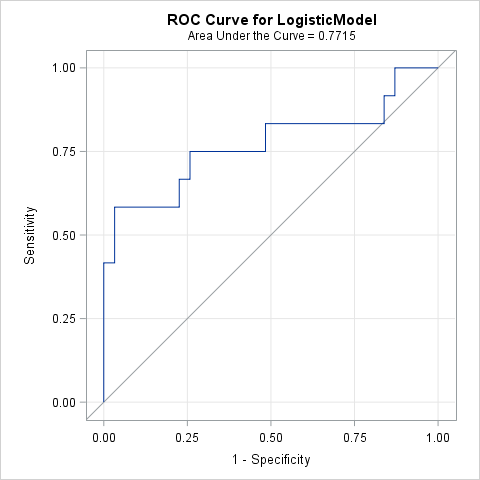You can see the documentation for details about how to interpret the output from PROC LOGISTIC, but the example shows that you can use the PLOTS=ROC option (or the ROC statement) to create an ROC curve for a model that is fit by PROC LOGISTIC. For this model, the area under the ROC curve is 0.77. Because a random "coin flip" prediction has an expected area of 0.5, this model predicts the survival of surgery patients better than random chance.

### Create an ROC curve for any prediction rule

A logistic model is not the only way to predict a binary response. You could also use a decision tree, a generalized mixed model, a nonparametric regression model, or even ask a human expert for her opinion. An ROC curve only requires two quantities: for each observation, you need the observed binary response and a predicted probability. In fact, if you carefully read the PROC LOGISTIC documentation, you will find these sentences:

• In the "Details" section: "ROC curves can be created ... from the specified model in the MODEL statement, from specified models in ROC statements, or from input variables which act as [predicted probabilities]." (Emphasis added.)
• In the documentation of the ROC statement: "The PRED= option enables you to input a criterion produced outside PROC LOGISTIC; for example, you can fit a random-intercept model by using PROC GLIMMIX or use survey weights in PROC SURVEYLOGISTIC, then use the predicted values from those models to produce an ROC curve for the comparisons."

In other words, you can use PROC LOGISTIC to create an ROC curve regardless of how the predicted probabilities are obtained! For argument's sake, let's suppose that you ask a human expert to predict the probability of each patient surviving for at least two months after surgery. (Notice that there is no statistical model here, only a probability for each patient.) The following SAS DATA step defines the predicted probabilities, which are then merged with the output from the earlier PROC LOGISTIC call:

```data ExpertPred; input ExpertPred @@; datalines; 0.95 0.2 0.05 0.3 0.1 0.6 0.8 0.5 0.1 0.25 0.1 0.2 0.05 0.1 0.05 0.1 0.4 0.1 0.2 0.25 0.4 0.7 0.1 0.1 0.3 0.2 0.1 0.05 0.1 0.4 0.4 0.7 0.2 0.4 0.1 0.1 0.9 0.7 0.8 0.25 0.3 0.1 0.1 ; data Survival; merge LogiOut ExpertPred; run;   /* create ROC curve from a variable that contains predicted values */ proc logistic data=Survival; model popind(event='0') = ExpertPred / nofit; roc 'Expert Predictions' pred=ExpertPred; ods select ROCcurve; run;```Notice that you only need to supply two variables on the MODEL statements: the observed responses and the variable that contains the predicted values. On the ROC statement, I've used the PRED= option to indicate that the ExpertPred variable is not being fitted by the procedure. Although PROC LOGISTIC creates many tables, I've used the ODS SELECT statement to suppress all output except for the ROC curve.

### Overlay and compare ROC curves from different models or rules

You might want to overlay and compare ROC curves from multiple predictive models (either from PROC LOGISTIC or from other sources). PROC LOGISTIC can do that as well. You just need to merge the various predicted probabilities into a single SAS data set and then specify multiple ROC statements, as follows:

```/* overlay two or more ROC curves by using variables of predicted values */ proc logistic data=Survival; model popind(event='0') = LogiPred ExpertPred / nofit; roc 'Logistic' pred=LogiPred; roc 'Expert' pred=ExpertPred; ods select ROCOverlay; /* optional: for a statistical comparison, use ROCCONTRAST stmt and remove the ODS SELECT stmt */ *roccontrast reference('Expert Model') / estimate e; run;```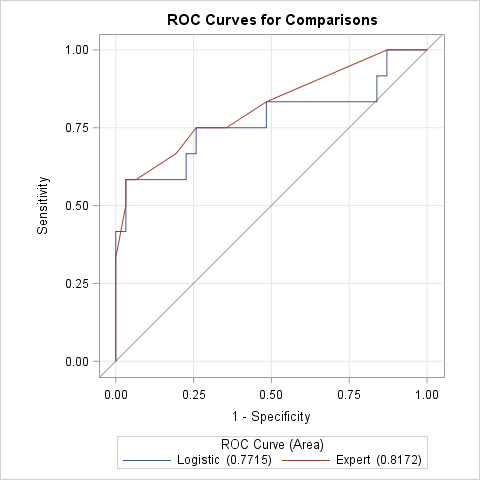This ROC overlay shows that the "expert" prediction is almost always superior or equivalent to the logistic model in terms of true and false classification rates. As noted in the comments of the previous call to PROC LOGISTIC, you can use the ROCCONTRAST statement to obtain a statistical analysis of the difference between the areas under the curves (AUC).

In summary, you can use the ROC statement in PROC LOGISTIC to generate ROC curves for models that were computed outside of PROC LOGISTIC. All you need are the predicted probabilities and observed response for each observation. You can also overlay and compare two or more ROC curves and use the ROCCONTRAST statement to analyze the difference between areas under the curves.

The post Create and compare ROC curves for any predictive model appeared first on The DO Loop.A frequent topic on SAS discussion forums is how to check the assumptions of an ordinary least squares linear regression model. Some posts indicate misconceptions about the assumptions of linear regression. In particular, I see incorrect statements such as the following:

• Help! A histogram of my variables shows that they are not normal! I need to apply a normalizing transformation before I can run a regression....
• Before I run a linear regression, I need to test that my response variable is normal....

Let me be perfectly clear: The variables in a least squares regression model do not have to be normally distributed. I'm not sure where this misconception came from, but perhaps people are (mis)remembering an assumption about the errors in an ordinary least squares (OLS) regression model. If the errors are normally distributed, you can prove theorems about inferential statistics such as confidence intervals and hypothesis tests for the regression coefficients. However, the normality-of-errors assumption is not required for the validity of the parameter estimates in OLS. For the details, the Wikipedia article on ordinary least squares regression lists four required assumptions; the normality of errors is listed as an optional fifth assumption.

In practice, analysts often "check the assumptions" by running the regression and then examining diagnostic plots and statistics. Diagnostic plots help you to determine whether the data reveal any deviations from the assumptions for linear regression. Consequently, this article provides a "getting started" example that demonstrates the following:

1. The variables in a linear regression do not need to be normal for the regression to be valid.
2. You can use the diagnostic plots that are produced automatically by PROC REG in SAS to check whether the data seem to satisfy some of the linear regression assumptions.

By the way, don't feel too bad if you misremember some of the assumptions of linear regression. Williams, Grajales, and Kurkiewicz (2013) point out that even professional statisticians sometimes get confused.

### An example of nonnormal data in regression

Consider this thought experiment: Take any explanatory variable, X, and define Y = X. A linear regression model perfectly fits the data with zero error. The fit does not depend on the distribution of X or Y, which demonstrates that normality is not a requirement for linear regression.

For a numerical example, you can simulate data such that the explanatory variable is binary or is clustered close to two values. The following data shows an X variable that has 20 values near X=5 and 20 values near X=10. The response variable, Y, is approximately five times each X value. (This example is modified from an example in Williams, Grajales, and Kurkiewicz, 2013.) Neither variable is normally distributed, as shown by the output from PROC UNIVARIATE:

```/* For n=1..20, X ~ N(5, 1). For n=21..40, X ~ N(10, 1). Y = 5*X + e, where e ~ N(0,1) */ data Have; input X Y @@; datalines; 3.60 16.85 4.30 21.30 4.45 23.30 4.50 21.50 4.65 23.20 4.90 25.30 4.95 24.95 5.00 25.45 5.05 25.80 5.05 26.05 5.10 25.00 5.15 26.45 5.20 26.10 5.40 26.85 5.45 27.90 5.70 28.70 5.70 29.35 5.90 28.05 5.90 30.50 6.60 33.05 8.30 42.50 9.00 45.50 9.35 46.45 9.50 48.40 9.70 48.30 9.90 49.80 10.00 48.60 10.05 50.25 10.10 50.65 10.30 51.20 10.35 49.80 10.50 53.30 10.55 52.15 10.85 56.10 11.05 55.15 11.35 55.95 11.35 57.90 11.40 57.25 11.60 57.95 11.75 61.15 ;   proc univariate data=Have; var x y; histogram x y / normal; run;```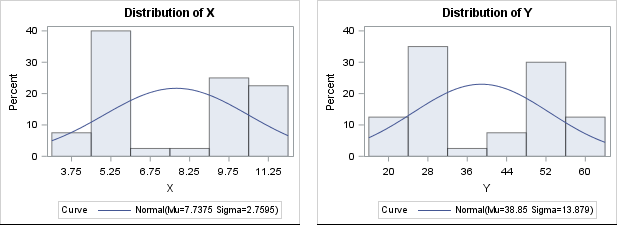There is no need to "normalize" these data prior to performing an OLS regression, although it is always a good idea to create a scatter plot to check whether the variables appear to be linearly related. When you regress Y onto X, you can assess the fit by using the many diagnostic plots and statistics that are available in your statistical software. In SAS, PROC REG automatically produces a diagnostic panel of graphs and a table of fit statistics (such as R-squared):

```/* by default, PROC REG creates a FitPlot, ResidualPlot, and a Diagnostics panel */ ods graphics on; proc reg data=Have; model Y = X; quit;```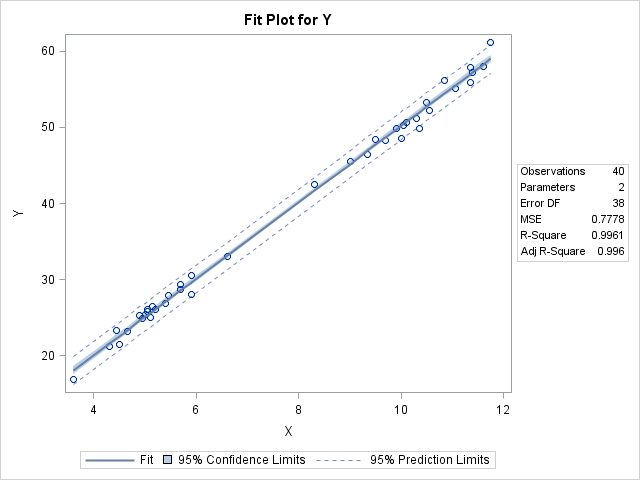The R-squared value for the model is 0.9961, which is almost a perfect fit, as seen in the fit plot of Y versus X.

### Using diagnostic plots to check the assumptions of linear regression

You can use the graphs in the diagnostics panel to investigate whether the data appears to satisfy the assumptions of least squares linear regression. The panel is shown below (click to enlarge).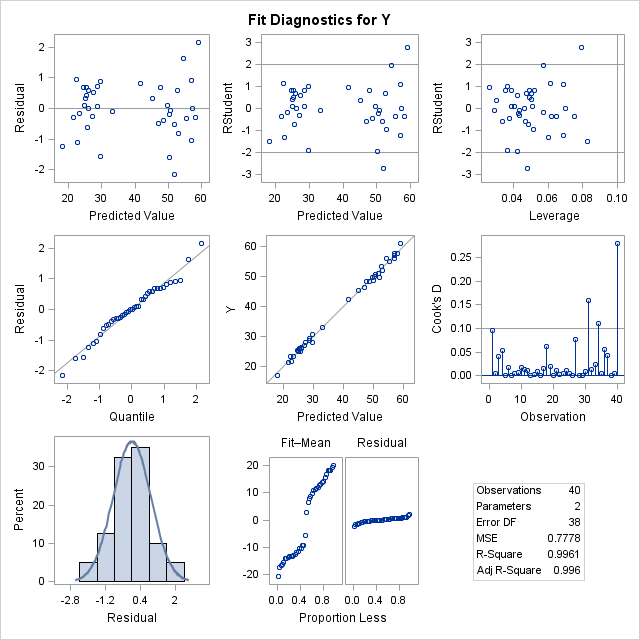The first column in the panel shows graphs of the residuals for the model. For these data and for this model, the graphs show the following:

• The top-left graph shows a plot of the residuals versus the predicted values. You can use this graph to check several assumptions: whether the model is specified correctly, whether the residual values appear to be independent, and whether the errors have constant variance (homoscedastic). The graph for this model does not show any misspecification, autocorrelation, or heteroscedasticity.
• The middle-left and bottom-left graphs indicate whether the residuals are normally distributed. The middle plot is a normal quantile-quantile plot. The bottom plot is a histogram of the residuals overlaid with a normal curve. Both these graphs indicate that the residuals are normally distributed. This is evidence that you can trust the p-values for significance and the confidence intervals for the parameters.